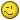# =SUBSTITUTE(;0;;1)

V

#### Vincent

Hi,

I am using the =SUBSTITUTE formula to extract "0" from
text string. It seems the formula is not working when
the "0" is neither the begin nor the end of the text
string. for example:
881050 =SUBSTITUTE(881050;0;;1)= 88150 instead of 88105.
Any idea why the above is not working?

Thanks,

L

#### Leo Heuser

Hi

The fourth argument (here 1) designates,
which instance will be replaced, so 1 will
replace the first instance (from left to right),
returning 88150, 2 will replace the second
instance, returning 88105 and leaving out
the fourth argument will replace all instances
thereby returning 8815.

G

#### Guest

I just need to replace the first and last instance.
How can I define that?
Could this work;=SUBSTITUTE(881050;0;;1;6)?

Thanks

A

I just need to replace the first and last instance.
How can I define that?
Could this work;=SUBSTITUTE(881050;0;;1;6)?
That would be an ambiguous substitution.
Give a set of example entries along with the desired results.

V

#### vincent

???
-----Original Message-----
Hi

The fourth argument (here 1) designates,
which instance will be replaced, so 1 will
replace the first instance (from left to right),
returning 88150, 2 will replace the second
instance, returning 88105 and leaving out
the fourth argument will replace all instances
thereby returning 8815.
--
Best Regards
Leo Heuser

"Vincent" <[email protected]> skrev i en meddelelse

.

H

#### Harlan Grove

Vincent said:
881050 =SUBSTITUTE(881050;0;;1)= 88150 instead of 88105.
Any idea why the above is not working?
It does work. You just don't know how to use it.

=SUBSTITUTE(881050;0;;1) means replace the first instance of 0 with nothing,
so delete it, thus getting 88150. If you want to delete the second instance
of 0, you must use =SUBSTITUTE(881050;0;;2), thus getting 88105.

P

#### Peo Sjoblom

=SUBSTITUTE(SUBSTITUTE(A1;0;;LEN(A1)-LEN(SUBSTITUTE(A1;0));0;;1)